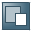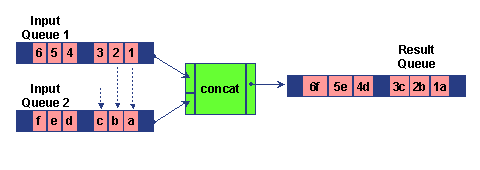Show TOC##Processing an Input Queue·        There is a queue for each field in the source structure.

·        Standard and user-defined functions process the input queues. The result of a calculation is written to a results queue.

·        The results queue for a function can in turn be an input queue for further functions.

·        Finally, there is also a queue for the target field. The restrictions for the target fields determine which of the values for the target field queue are written to the target structure.

If you have created an XML instance in the test environment editor, you can display the various queues in the data-flow editor.

#### Input Queues of Varying Lengths

If the source structure contains elements with an occurrence value greater than 1, then one context in a queue may contain multiple values. Provided that the input queues have the same number of values for each context, it is clear how the function processes the values:However, in the following example, the input queues for the concat function have a different number of values in the contexts that belong together. If, as in this case, an input value is missing in a queue, the message mapping runtime simply uses the last value that it read from the queue in question.

The same applies for generating functions (constants, for example) that only generate one value if they are connected with a function: If another input queue has more than one value in a context, the mapping runtime simply uses the value generated by the generating function again.